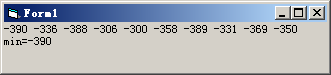# VB程序题：编一子过程 ProcMin(a()),amin),求一维数组a中的最小值amin VB源码 龚沛曾

VB程序题：编一子过程 ProcMin(a()),amin),求一维数组a中的最小值amin  VB源码 龚沛曾

Private Sub Form_Click()
Dim a(1 To 10), amin, i%
Randomize
For i = 1 To 10
a(i) = -Int(Rnd * 101 + 300)
Print a(i);
Next i
Call s(a(), amin)
Print
Print "min="; amin
End Sub
Sub s(b(), min)
Dim i%
min = b(LBound(b))
For i = LBound(b) + 1 To UBound(b)
If b(i) < min Then min = b(i)
Next i
End SubVisual Basic程序设计教程(第3版) (龚沛曾等编)课后实验源码

<< 上一篇 下一篇 >>